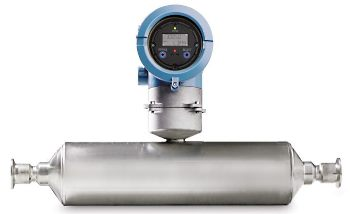calculator.org

# property>molar flowrate

## What is Molar Flowrate?Molar flowrate is a measure of the amount of substance (the number of molecules) that passes through a given area perpendicular to the flow in a given time. Typically this area is constrained, for example a section through a pipe, but it could also apply to an open flow.

If the velocity can be measured, and since the density and cross-sectional area are usually known, the molar flow rate can be calculated from the product of the area, the density and the velocity, divided by the molecular weight. It can also be computed by taking the volumetric flowrate and multiplying it by density and dividing by the molecular weight.

Determining molar flowrates is important in chemical engineering in order to control reaction rates in reactor vessels.

Bookmark this page in your browser using Ctrl and d or using one of these services: (opens in new window)